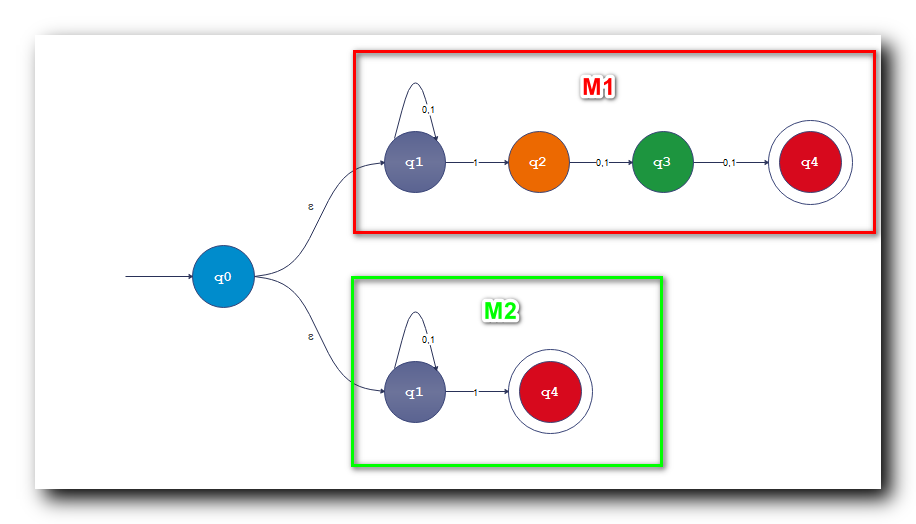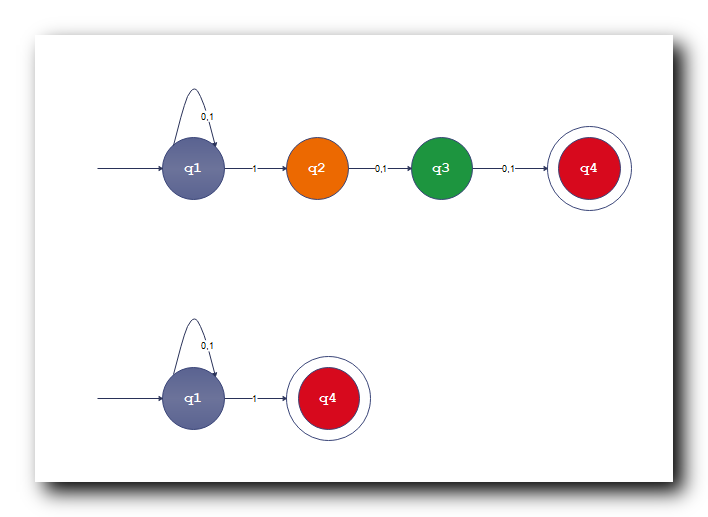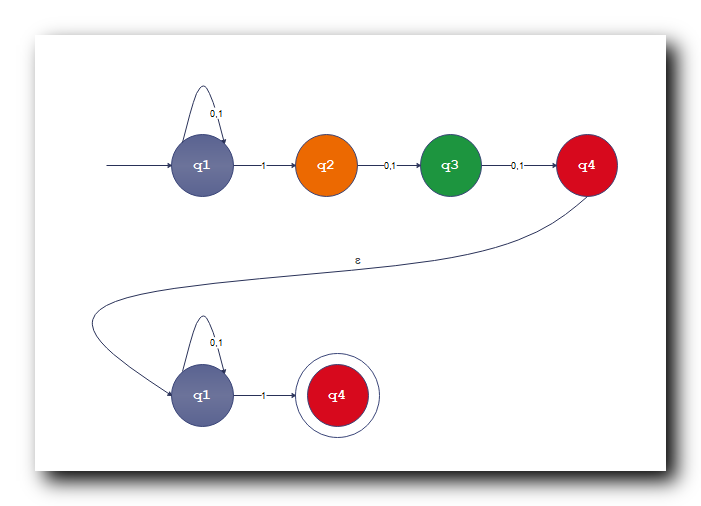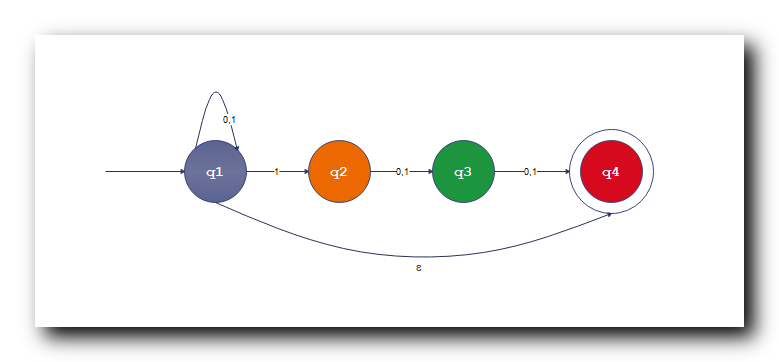# 一、正则语言引入

1 . 非确定性有限自动机 作用 : 非确定性有限自动机并没有增加 自动机 的计算能力 , 但是给自动机设计带来很多方便 ; 仅限于在理论计算时带来很多方便 , 但是无法实现 ;

2 . 自动机实现 : 非确定性有限自动机 ( NFA ) 的的优点是给自动机的设计带来了很多方便 , 但是 只有 确定性的有限自动机 ( DFA ) 才能被实现出来 ;

3 . 自动机等价 : 通过算法可以判定两个确定性的有限自动机是等价的 ,

4 . 自动机优化 : 给定确定性有限自动机 , 可以将该自动机优化 , 得到一个最小的与该 DFA 等价的 自动机 ;

5 . 自动机涉及的两个问题 :

① 优化问题 : 给定一个自动机 , 如何找到一个算法 , 将自动机最小化 ;

② 设计自动机 : 给定一个语言 , 如何找到一个算法 , 根据该语言设计出自动机 ;

6 . 引入正则语言 : 确定性有限自动机 ( DFA ) 与 非确定性有限自动机 ( NFA ) 接受的是相同的语言 , 这个语言就是正则语言 ;

# 二、正则语言

( 这个自动机可以是 确定性有限自动机 , 也可以是 非确定性有限自动机 ; )

① 自动机设计要求 : 给定一个语言 , 设计能识别该语言的自动机 ;

② 算法自动设计 : 自动机设计的过程 , 有的很复杂 , 希望能找到一个算法 , 使用该算法实现 自动机的设计 ;

③ 语言特点 : 如果要设计能识别 某语言的自动机 , 那么需要先了解这个语言有什么特点 , 知道这个语言的特点就可以设计 识别该语言的自动机 ;

# 三、 正则语言运算 ★

1 . 并运算 ( Union ) : A , B A, B 两个语言并在一起 , 就是将 A A 语言的字符串 , 和 B B 语言的字符串并在一起就可以 ;

A ∪ B = { x ∣ (    x ∈ A    ) ∨ (    x ∈ B    ) } \rm A \cup B = \{ \quad x \quad | \quad ( \; x \in A \; ) \lor ( \; x \in B \; ) \quad \}

2 . 串联运算 ( Concatenation ) : A A 语言的字符串 与 B B 语言中的字符串串在一起 , 注意 A A 语言字符串在前 , B B 字符串在后 ;

A ∘ B = { x y ∣ (    x ∈ A    ) ∧ (    x ∈ B    ) } \rm A \circ B = \{ \quad xy \quad | \quad ( \; x \in A \; ) \land ( \; x \in B \; ) \quad \}

3 . 星运算 ( Star ) :

A ∗ = { x 1 x 2 ⋯ x k ∣ x i ∈ A } ( 0 ≤ i ≤ k ) \rm A^*= \{ \quad x_1 x_2 \cdots x_k \quad | \quad x_i \in A \quad \} \quad\quad (0 \leq i \leq k)

• 计算本质 : 计算的实质是循环 , 在现实中的计算 , 其本质也是不停的重复循环 , 进行计算 ;

• 计算机作用 : 计算机可以代替重复的计算 ;

• 循环运算抽象 : 星运算实质上是对循环运算的抽象表述 ;

• 自动机计算 : 在有限自动机中 , 可以做循环计算 , 使用 星 计算 实现 循环计算 ;

( 有限个字符串的 有限个 是不确定的值 , 可以是 10万 , 100亿 ⋯ \cdots )

# 四、语言运算示例 ★

A A 语言 : A = { 001 , 10 , , 111 } A = \{ 001 , 10, , 111 \}

B B 语言 : B = { ε , 001 } B = \{ \varepsilon , 001 \}

1 . 并计算 : A , B A,B 两个语言的集合 , 取 并集 即可 , 计算如下 :

A ∪ B = { 001 , 10 , 111 , ε } \rm A \cup B = \{ 001, 10 , 111 , \varepsilon \}

2 . 串联计算 : A A 语言中的任意字符串 , 与 B B 语言中的任意字符串 , 串联在一起 , A A 语言中有 3 3 个字符串 , B B 语言中有两个字符串 , 那么串联的结果有 2 × 3 = 6 2 \times 3 = 6 个 , 计算过程如下 :

A ∘ B = { 001 , 10 , 111 , 001001 , 10001 , 111001 } \rm A \circ B = \{ 001 , 10, 111 , 001001, 10001, 111001 \}

3 . 星计算 : A A 语言的星计算 , 该集合一定是一个无限的集合 , 如果 A A 语言不是空集 , 那么该 A ∗ A^* 集合个数是无限的 , 其可以由 K K 个字符串组成 , K K 取值 0 0 到无穷大 , [ 0 , + ∞ ) [0 , +\infty ) ;

A ∗ = { ε , 001 , 10 , 111 , ⋯   } \rm A^* = \{ \varepsilon , 001 , 10 , 111 , \cdots \}

# 五、正则语言封闭性 ★

A , B A, B 两个语言是正则语言 , 那么 A ∪ B A \cup B , A ∘ B A \circ B , A ∗ A^* 都是正则语言 ;

A ∪ B A \cup B 语言证明 :

① 已知自动机 与 语言 : 假设有自动机 M 1 M_1 识别语言 A A , 自动机 M 2 M_2 识别语言 B B ;

② 设计的 新自动机 与 语言 : 设计新的自动机 M M 识别语言 A ∪ B A \cup B , A ∘ B A \circ B , A ∗ A^* ;

# 六、正则语言封闭性 A ∪ B A \cup B 证明

A ∪ B A \cup B 语言证明 :

① 无条件跳转 : 引入一个新的状态 , 这个新的状态 , 接受 ε \varepsilon 即可跳转到 M 1 M_1 M 2 M_2 的开始状态 ;

② 新自动机 : 自动机 M M 所接受的语言 , 实际上就是 M 1 M_1 自动机所接受的语言 A A M 2 M_2 自动机所接受的语言 B B 的并集 , 即 A ∪ B A \cup B ;# 七、正则语言封闭性 A ∘ B A \circ B 证明

A ∘ B A \circ B 语言证明 :

① 旧的自动机与语言 : 假设有自动机 M 1 M_1 识别语言 A A , 自动机 M 2 M_2 识别语言 B B ;

② 新的自动机与语言 : 设计新的自动机 M M 识别语言 A ∘ B A \circ B ;# 八、正则语言封闭性 A ∗ A^* 证明

A ∗ A^* 语言 封闭性 证明 : 一个自动机 M M 识别 A A 语言 , 获取识别 A ∗ A^* 语言的自动机 , 只需要将 该自动机 M M 的开始状态 与 接受状态 连接起来即可 ;# 九、自动机扩展

### echarts x轴数据过多左右滚动显示_echarts x轴滚动-程序员宅基地

echarts x轴数据过多左右滚动显示今天在使用echarts做柱状图时，遇到x轴数据过多的情况，全部显示的话，柱状图都挤到一起了。所以想让它可以显示一部分，其余部分滚动显示，就可以配置dataZoom，它与xAxis、yAxis并列，简单配置后，就可以实现x轴刻度左右滚动了。代码如下：dataZoom: [ { type: "slider", show: true, xAxisIndex: , _echarts x轴滚动

### innodb_flush_method及O_DIRECT参数详解与测试_mysql error while setting value 'o_direct' to 'inn-程序员宅基地

https://blog.csdn.net/smooth00/article/details/72725941_mysql error while setting value 'o_direct' to 'innodb-flush-method'.

### 【2021山东大学数字逻辑实验4】 译码器_4线16线译码器真值表_jinzhou742的博客-程序员宅基地

1、实验内容本实验要求完成一个3线－8线译码器的设计。其中i2-i0为译码器输入端，y7-y0为译码器输出端。图3.7为三线―八线译码器的框图，图8给出了三线―八线译码器的原理图。图3.7 3线―8线译码器框图:2、实验原理图①3-8译码器：②4-16译码器：引脚分配图：键K4是使能端，键K3~ K0控制4位二进制数的读入，小灯LR15~LR0控制译码结果的显示。如果要使用3-8译码器，则只需使用键K1和K0即可。该译码器为4-16译码器，设计思路是根据输入输出的真值表来连线，逻辑比_4线16线译码器真值表